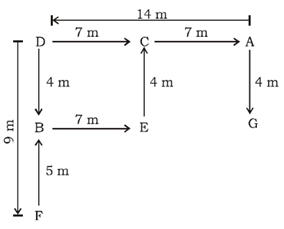# SSC CGL 2018 Practice Test Papers | Reasoning Ability (Day-25)

Dear Aspirants, Here we have given the Important SSC CGL Exam 2018 Practice Test Papers. Candidates those who are preparing for SSC CGL 2018 can practice these questions to get more confidence to Crack SSC CGL 2018 Examination.

[WpProQuiz 3035]

Click “Start Quiz” to attend these Questions and view Explanation

1)  X is the father of Y and Z. Y is the son of X, but Z is not the son of X. How is Z related to Y?

a) Mother

b) Aunt

c) Wife

d) Sister

2) If ‘+’ is ‘÷’, ‘–’ is ‘×’, ‘×’ is ‘+’ and ‘÷’ is ‘–’, then what will be the value of 36 + 12 – 6 × 2 ÷ 10?

a) 25

b) 10

c) 30

d) 15

3) Which of the following is different from the others?

a) DKM

b) TAC

c) BIK

d) YFI

4) If CRACK is coded as 15718 and WICKET is coded as 231869, what will be the code for CRICKET?

a) 1534896

b) 356289

c) 1531869

d) 2356741

5) Select the related word from the given alternatives

TALE : LATE : : ? : CAFÉ

a) FACE

b) FECA

c) CAFÉ

d) EFAC

6) Find the odd one.

a) Torch

b) Candle

c) Moon

d) Sun

7) Three positions of a dice are shown below. Which letter will be opposite to ‘O’?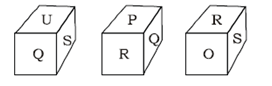a) Q

b) S

c) U

d) P

D is 14 meters west of A. B is 4 meters south of D. F is 9 meters south of D. E is 7 meters east of B. C is 4 meters north of E. G is 4 meters south of A.

8)If a person goes 5 metERS from F in north direction and then turn right, then he will reach which of the following point first?

a) G

b) C

c) A

d) E

9) What is the distance between points D and A?

a) 14 m

b) 10 m

c) 9 m

d) 4 m

10) Point F is in which direction with respect to Point A?

a) North west

b) South west

c) North East

d) Southeast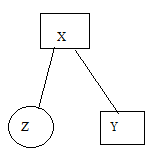36 ÷ 12 x6 +2 –10 =10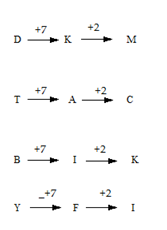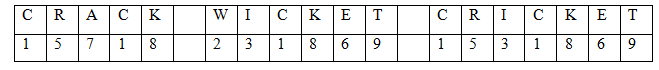The position of first and third letters are interchanged.

Except at all others are natural source of light.

Adjacent faces of S are R, U, O and Q

The face opposite to S is P.

From the diagram I and III it is clear that U and R are opposite to each other.

Therefore the face opposite to O is Q.